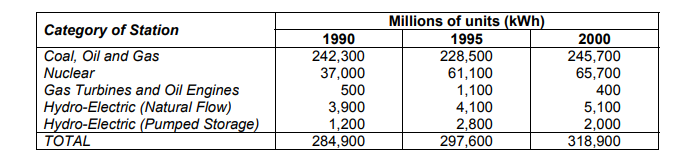# IMAT 2011 Q23 [Electricity Generation]

The table below relates to electricity generation in the United Kingdom:Which category of station made the largest contribution to the increase in total units generated over the 10 years covered by the table?

A. Coal, Oil and Gas
B. Nuclear
C. Gas Turbines and Oil Engines
D. Hydro-Electric (Natural Flow)
E. Hydro-Electric (Pumped Storage)

Some Quick Questions to ask yourself when doing this type of problem solving:

• What is being asked? Underline the specifics in the question.
• Analyse the data, is there anything we can eliminate immediately?
• What information do I need to solve the question? Where can I find it?
• How can I draw or use the graph to help me?

This question can be overwhelming at first but it is testing your ability to spot outliers so you only really have to do 1-2 calculations. Lets explain by going through each option. Tip: read in reverse order, this is because the bottom numbers are small and less likely to be the greatest contributor since they change only a bit. Also note that it is asking for what contributes the greetes over a 10 year period, therefore we can neglect any answers that end up increasing but then finishing at a value lower than the original one.

A This option can be tricky and it tests your comprehension of the question. Note that we are looking for what is contributing the greatest change over a 10 year period, so we will take the difference between 1990’s output and 2000’s output. This is important because this answer fluctuates in the middle. If we take the difference between 2000 and 1990, we see that the kWh is only increased by 3400kWh.

B This answer shows a big jump from 1990 to 1995, but a smaller one from 1995 to 2000. This does not matter, we are looking at the increase over a 10 year period, so we will find the difference in kWh from 1990 to 2000. This amounts to be 28700kWh, which is the greatest of any option. Therefore it is the correct answer.

C This is the easiest answer to eliminate. It is the only option having a net decrease over the 10 year period. If you understand the question (i.e know that it is over 10 years) then this should be the first one you eliminate.

D Hydro-Electric (natural storage) is on a steady increase, however it is still only going up 1200. When dealing with these smaller numbers, you don’t even need to calculate the exact amount because we can tell it will be either A or B. As a result eliminate these distractor options quickly so you have time to calculate if needed.

E Hydro-Electric (pumped storage) goes up 1600 but then down 800 again. This answer can easily be eliminated as the numbers are fluctuating small amounts. Just eyeballing the difference in total, we can tell that there is an increase in tens of thousands of kWh, so a change of 800 won’t be a huge contributor.